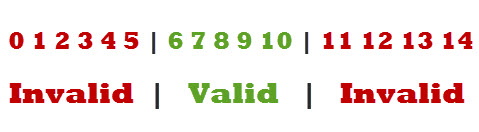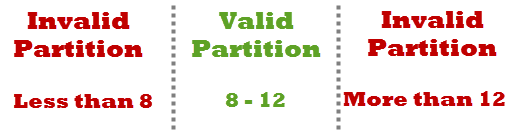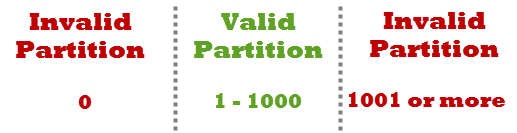## 10/17/15

### Input domain testing (ECP, BVA)

Boundary value analysis and Equivalence Class Partitioning both are test case design techniques in black box testing. In this article we are covering “What is Boundary value analysis and equivalence partitioning & its simple examples”.

## What is Equivalence Class Partitioning?

Equivalence partitioning is a Test Case Design Technique to divide the input data of software into different equivalence data classes. Test cases are designed for equivalence data class. The equivalence partitions are frequently derived from the requirements specification for input data that influence the processing of the test object. A use of this method reduces the time necessary for testing software using less and effective test cases.

Equivalence Partitioning = Equivalence Class Partitioning = ECP
It can be used at any level of software for testing and is preferably a good technique to use first. In this technique, only one condition to be tested from each partition. Because we assume that, all the conditions in one partition behave in the same manner by the software. In a partition, if one condition works other will definitely work. Likewise we assume that, if one of the condition does not work then none of the conditions in that partition will work.
Equivalence partitioning is a testing technique where input values set into classes for testing.
• Valid Input Class = Keeps all valid inputs.
• Invalid Input Class = Keeps all Invalid inputs.

## Example of Equivalence Class Partitioning?

• A text field permits only numeric characters
• Length must be 6-10 characters long
Partition according to the requirement should be like this:While evaluating Equivalence partitioning, values in all partitions are equivalent that’s why 0-5 are equivalent, 6 – 10 are equivalent and 11- 14 are equivalent.
At the time of testing, test 4 and 12 as invalid values and 7 as valid one.
It is easy to test input ranges 6–10 but harder to test input ranges 2-600. Testing will be easy in the case of lesser test cases but you should be very careful. Assuming, valid input is 7. That means, you belief that the developer coded the correct valid range (6-10).

## What is Boundary value analysis:

Boundary value analysis is a test case design technique to test boundary value between partitions (both valid boundary partition and invalid boundary partition). A boundary value is an input or output value on the border of an equivalence partition, includes minimum and maximum values at inside and outside boundaries. Normally Boundary value analysis is part of stress and negative testing.
Using Boundary Value Analysis technique tester creates test cases for required input field. For example; an Address text box which allows maximum 500 characters. So, writing test cases for each character once will be very difficult so that will choose boundary value analysis.

## Example for Boundary Value Analysis:

Example 1
Suppose you have very important tool at office, accepts valid User Name and Password field to work on that tool, and accepts minimum 8 characters and maximum 12 characters. Valid range 8-12, Invalid range 7 or less than 7 and Invalid range 13 or more than 13.Write Test Cases for Valid partition value, Invalid partition value and exact boundary value.
• Test Cases 1: Consider password length less than 8.
• Test Cases 2: Consider password of length exactly 8.
• Test Cases 3: Consider password of length between 9 and 11.
• Test Cases 4: Consider password of length exactly 12.
• Test Cases 5: Consider password of length more than 12.

Example 2
Test cases for the application whose input box accepts numbers between 1-1000. Valid range 1-1000, Invalid range 0 and Invalid range 1001 or more.Write Test Cases for Valid partition value, Invalid partition value and exact boundary value.
• Test Cases 1: Consider test data exactly as the input boundaries of input domain i.e. values 1   and 1000.
• Test Cases 2: Consider test data with values just below the extreme edges of input domains i.e. values 0 and 999.
• Test Cases 3: Consider test data with values just above the extreme edges of input domain i.e. values 2 and 1001.

### Over to you on Boundary Value Analysis and Equivalence Class Partitioning:

Till now we have seen about What is Boundary value analysis and equivalence partitioning & its simple examples. Here we have covered very basic and simple example to understand the most commonly used test case design techniques. There is no such hard and fast rule to  take only one input from each partition. Based on your needs and previous experience you can decide the inputs.